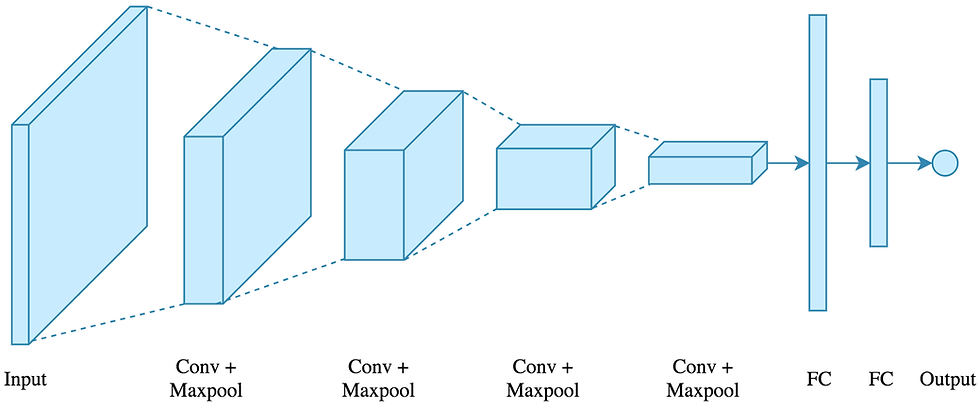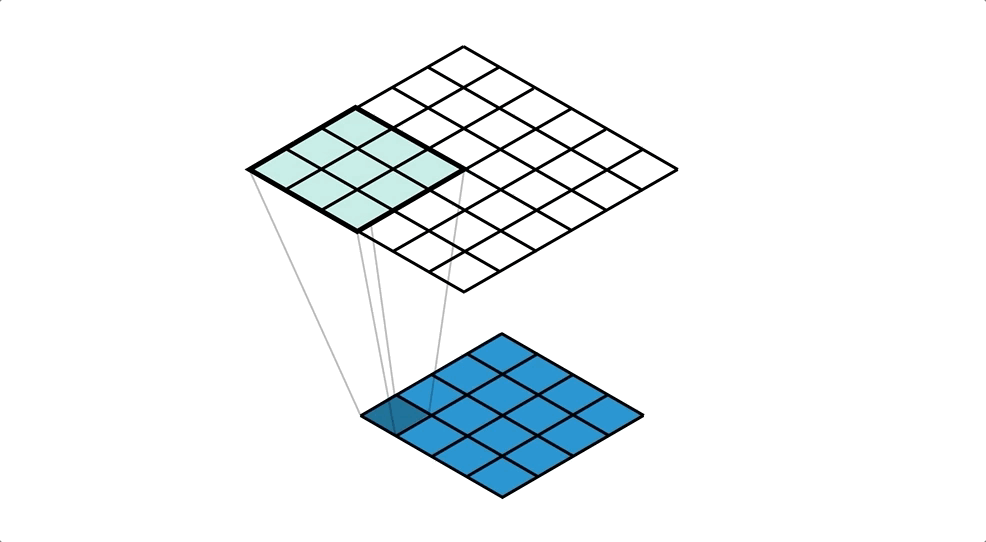top of page

# What is Deep Learning? An intuitive introduction

Updated: Apr 19

### It’s much easier than what you think it isIn recent days, there has been a lot of improvements in this modern technological world like autonomous cars, facial recognition systems, chatbots to name a few. These cool inventions are made possible only with the help of Deep Learning. Read this article to know what deep learning is and its supporting factors that made deep learning so popular.

## Deep Learning

Deep learning is a subset of Machine Learning (ML) that gives us certain predictions for the given set of inputs by learning from examples or previous data points. That’s what the whole concept is about. Technically speaking, deep learning is the higher form of Machine Learning that replicates the working of the human brain to perform complex tasks. Deep learning is much powerful than Machine Learning in that it is capable of giving accurate predictions for both structured (data that has columns and rows, for example, housing data, etc.) and unstructured data (examples are audio patterns, images, etc.). After knowing the concept of deep learning, it suddenly leads us to the so-called concept of Artificial Neural Networks (ANN) or just Neural Networks (NN) which explains the mechanism of deep learning.

## Neural Networks

Before diving into the concept of neural networks, let’s imagine a scenario and observe how the human body acts to it. Imagine that you keep your hand on a burning fire. Within a fraction of a second, the sensory organs present in your hands transmit the signal to the brain via the so-called neurons. The human brain then sends the message to take our hands off the fire again via the neurons and finally we take our hands off the fire. The main agent that acts as an important unit in this scenario is the neurons. The collection of human neurons are called as Biological Neural Network. This same concept of Biological Neural Network applies to Artificial Neural Networks.

To understand the concept better, let’s tackle the same situation but with Artificial Neural Networks. Let’s take, the signal obtained when touching the fire as the input. The input is then processed by a similar structure of neurons called the units. Instead of the brain, the units take the optimal decision and decide whether to take the hands off the fire or not (0/1).

Technically speaking, Neural Networks are a replication of Biological Neural Networks that filters the input using layers of units and gives accurate predictions. Each unit is interconnected to each other, thus forming a network. The structure of a Neural Network looks like this:As you can see, the neural network is divided into an input layer, the hidden layer(s), and an output layer.

Input layer: This layer contains the data points of the data which is being inserted into the neural network.

Hidden layer(s): This is the most important layer of the neural network as all the functions and processes take place in this layer. Each layer is activated by the so-called activation functions like ReLU (Rectified Linear Unit), Sigmoid (Probabilistic Curve), and Softmax.

Output layer: As the name suggests, this layer shows the final output of the given set of inputs after a series of processings.

## Types of ANN

There are a total of seven types of artificial neural networks but here we are going to discuss only the most famous two types of ANN. They are Convolutional Neural Network (CNN), and Recurrent Neural Network (RNN).

### Convolutional Neural Network (CNN)

Convolution Neural Network is a type of neural network that is most commonly applied to analyze and tackle visual imagery problems. Just like how Neural Networks are inspired by the design and functionality of the human brain, CNN resembles the architecture of the human or animal visual cortex. The architecture of CNN looks like this:Convolution layer (Conv): The convolutional layers perform convolution operations with the help of filters and produces an output called feature map or activation map. The convolution operation that takes place inside the convolution layer looks like this:Pooling layer (Maxpool): The pooling layer in CNN acts as an agent in the reduction of the spatial size which ultimately leads to the reduction of the computation of the network. It performs its function on every feature map or activation map independently. The pooling layer can be classified into two types they are Average pooling and max pooling. The most commonly used pooling function is the Max pooling function (that is what used in the illustration too). Max pooling calculates and selects the largest value in each patch in each feature map that is separated by filters. On the other hand, Average pooling is nothing but it calculates and selects the average value in each patch in each feature map that is separated by filters. The calculation performed by the Max pooling function looks like this:Fully Connected layer (FC): After pooling the feature map into a matrix, it is converted into a single long feature of vectors or a one-dimensional array for inputting it to the next further layers. This process is called flattening. Then, it is connected to the final model called the Fully Connected layer. In most of the models, the Fully Connected layers are present at the end of the network.

Applications of CNNs are image classification, face recognition, human pose estimation to name a few.

### Recurrent Neural Network (RNN)

Recurrent Neural Networks are primarily used to deal and build models for sequential data. It plays a major role in solving Natural Language Processing (NLP) tasks. Recurrent Neural Networks are also primarily used for time series analysis and forecasting. The most popular type of Recurrent Neural Network is the Long Short Term Memory (LSTM) algorithm. There are a total of five types of RNN are (Note: Tx = Number of Inputs, Ty = Number of Outputs):

• One-to-one (Tx = Ty = 1). Example: Traditional Neural Network

• One-to-many (Tx = 1, Ty > 1). Example: Music Generation

• Many-to-one (Tx > 1, Ty = 1). Example: Sentiment Analysis

• Many-to-many (Tx = Ty). Example: Name Entity Recognition

• Many-to-many (Tx is not equal to Ty). Example: Machine Translation

The foremost important advantage of RNN is that they have the ability to build models for sequential data which is called time series and this advantage is considered the most important because for time series analysis each sample values are dependent on the previous ones. The biggest drawback of RNN is the fact their computational time is really slow.

## Why is Deep Learning taking off?

The concept and mathematics behind deep learning and neural networks are invented back in 1943 but, no one ever knew such a concept even existed or not until the recent years of 2000 and ahead. The real surge in the use of deep learning as a tool to solve problems is in and after the year 2010. Why is it so? The driver of deep learning progress is the vast amount of data that we have at present. Decades earlier, we did have data but on a very small scale, and when the traditional ML algorithms like SVM, Logistic Regression applied to these small scale data, they were not able to perform significantly. This case was not there in the last few years. In the past ten years, the increment of digitization, and people’s usage of electronic gadgets helped in collecting and storing a vast amount of data. Along with that, the tremendous algorithmic inventions with strong computation, helped deep learning algorithms to provide results that are far more than the traditional ML algorithms. So, we can simply say:

### 'Scale drives Deep Learning progress'

You might ask, what if there isn’t a significant amount of data? The answer is, then deep learning won’t exist. That’s the only disadvantage of deep learning.

## Final Thoughts!

Hope you enjoyed this article. In this article, we covered almost all the basic foundations of deep learning but there is still a lot to explore. The math part of neural networks and their types might a bit tough but never hesitate to touch upon it. The most interesting part of deep learning is its practical implication and of course, coding. With help of deep learning, we can be able to create awesome and cool applications like image detection, forecasting, and many more wonderful things. I will guide you through the coding part in my upcoming posts and follow me to never miss any of them.

Happy Deep Learning!

Tags: